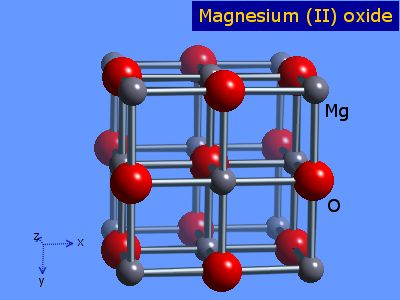# Question #95b54

Mar 18, 2015

Sodium fluoride, $N a F$, is isomorphous with magnesium oxide, $M g O$, because their crystal structures are very similar in shape.

Sodium fluoride and magnesium oxide are actually isosteric compounds, i.e. they have the same number of valence electrons, but they differ in the type of atoms that form them.

Because the cations and anions that form these two compounds differ by just 1 unit of charge ($N {a}^{+}$, $M {g}^{2 +}$, and ${F}^{-}$, ${O}^{2 -}$), they crystalize in a similar face-centered cubic structure.As you can see, the interionic distances are shorter in the case of $M g O$. This happens because of the greater electrostatic attraction that holds these ions together (greater magnitude of charge, greater electrostatic attraction).

Mar 18, 2015

They are isomorphous because the ratio of the radii of their cations and anions $\left({r}^{+} / {r}^{-}\right)$ lies within the range which predicts a face centred cubic structure.

Ionic bonds are non - directional and an ionic solid will consist of a symmetrical array of positive and negative ions.

They will form a structure that ensures a minimum amount of energy, hence maximum stability.

If cations are equal in size to anions each cation would touch 12 anions and vice versa. The coordination number is said to be 12.

In reality cations tend to be smaller than anions so it is not possible to "fit" 12 anions around them so coordination numbers tend to be less than 12.

It is the ratio of the cation to anion radius ${r}^{+} / {r}^{-}$ which decides the most stable arrangement.

By doing some geometry (which I won't go into here) it can be shown that if ${r}^{+} / {r}^{-}$ lies between 0.732 and 0.414 for a binary compound then a cubic structure where each cation is in contact with 6 anions and vice versa results:

For $M {g}^{2 +} , {r}^{+} = 0.065 \text{nm}$

For ${O}^{2 -} , {r}^{-} = 0.140 \text{nm}$

So ${r}^{+} / {r}^{-} = \frac{0.065}{0.140} = 0.464$

Since this lies within the range stated it forms this structure:The "bonds" are actually points of contact. If you look at the ${O}^{2 -}$ ion at the centre of the cube you can see it is touching 6 $M {g}^{2 +}$ ions. They in turn are touching 6 ${O}^{2 -}$ ions. This is 6:6 coordination.

There is an ion at the centre of the face of the cube, so this structure is referred to as "face - centred cubic".

For $N a F$:

${r}^{+} = 0.095 \text{nm}$

${r}^{-} = 0.136 \text{nm}$

${r}^{+} / {r}^{-} = \frac{0.095}{0.136} = 0.67$

Since this value is between 0.414 and 0.732 we can also predict a face - centred cubic structure.